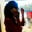Related Tags

c
communitycreator

# How to implement merge sort in CSmriti Mallick

Merge Sort is one of the most efficient sorting algorithms based on the principle of the Divide and Conquer Algorithm. Basically, it divides a given list into several sub-lists; then, merge those sub-lists recursively until there is only one sub-list left (i.e., the sorted list).

This is one of the best sorting techniques for sorting Linked Lists.

1 of 17

## Time complexity of merge sort

The time complexity of a merge sort algorithm is, $O(nlog \space n)$ .

The time complexity is the same for all three casesworst case, average case, and best case. This is because merge sort divides the given array of elements into two halves, taking the linear time to merge the two halves.

The space required for sorted and unsorted arrays are the same. Therefore, it is not preferable to searching large, unsorted arrays.

## Code

The code below follows the of divide and conquer principle: it takes the list and starts to split the given list into equal halves (i.e., sub-lists). This process is continued recursively until we only have one element in each sub-list.

Then, merge() starts combining two sub-lists at a time in ascending order, placing the lower element to the left of the list. This process continues until we only have one sub-list left, i.e., the sorted array.

#include<stdio.h>
#include<time.h>
#include<stdlib.h>

// function to merge the sub-arrays
void merge(int a[],int low,int mid ,int high)
{
int b; //same size of a[]
int i,j,k;
i=low,j=mid+1,k=low;
while(i<=mid && j<=high)
{
if(a[i]<=a[j])
b[k++]=a[i++];
else
b[k++]=a[j++]; //copying the elements
}
while (i<=mid)
b[k++]=a[i++];
while
(j<=high) b[k++]=a[j++];
for (k=low;k<=high;k++)
a[k]=b[k];
}

// merge sort function
void mergesort(int a[],int low,int high)
{
int mid;
if(low>=high)
return;
mid=(low+high)/2;
mergesort(a,low,mid);
mergesort(a,mid+1,high);
merge(a,low,mid,high);
}

// main fucntion
int main()
{
int a = {83, 20, 9, 50, 115, 61, 17};
int n = 7;

mergesort(a, 0, n-1);

printf("\n Sorted numbers are : \n");

// function to print the sorted array
int k;
for(k=0;k<7;k++)
printf("%d\t",a[k]);
return 0;
}


RELATED TAGS

c
communitycreator

CONTRIBUTORSmriti Mallick
RELATED COURSES

View all Courses

Keep Exploring

Learn in-demand tech skills in half the time